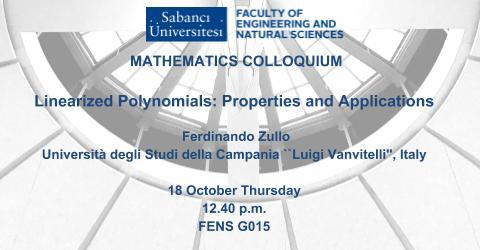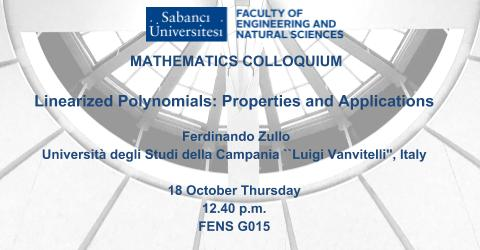## Mathematics Colloquium: Linearized Polynomials: Properties and Appli...

### Mathematics Colloquium: Linearized Polynomials: Properties and Appli...Speaker: Ferdinando Zullo

Title: Linearized Polynomials: Properties and Applications

Date/Time: October 18, 2018  /  12:40-13:30

Place: FENS G015

Abstract: Linearized polynomials over \$\F_{q^n}\$ are important objects in the theory of finite fields and in finite geometry since they correspond to \$\F_q\$-linear transformations of the \$n\$-dimensional \$\F_q\$-vector space \$\F_{q^n}\$, and can be used to describe related objects such as \$\F_q\$-subspaces, rank metric codes and \$\F_q\$-linear sets.

The characterization of the number of roots of a linearized polynomial in the coefficients field directly from their coefficients is an important tool in the applications.

With Bence Csajb\'ok, Giuseppe Marino and Olga Polverino we investigate this problem providing sufficient and necessary conditions for the coefficients of a linearized polynomial over \$\F_{q^n}\$ ensuring that the number of its distinct roots is the maximum possible.

Furthermore, we will deal with some applications of these results.

Contact: Michel Lavrauw